## 位图基础 & 图像显示

### 图像和图形区别

• 图形是指令的集合，比如 Point1.LineTo(Point2)
• 图像是一个数值矩阵。

### 索引图像 vs 真色彩图像

• 索引图像，有颜色表调色板索引。矩阵存储的是索引号。
• 真色彩图像，矩阵直接存储色彩像素信息。

UltraEdit 软件。基本的编辑文本、十六进制、ASCII 码、语法加亮、代码折叠、代码单词拼写检查等。

## 图像几何变换

### 齐次坐标

https://zhuanlan.zhihu.com/p/80852438

### 仿射变换 & 透视变换

#### 仿射变换（Affine Transformation 或 Affine Map）

$\left\{\begin{array}{l}u=a_{1} x+b_{1} y+c_{1} \\v=a_{2} x+b_{2} y+c_{2}\end{array}\right.$ $\left[\begin{array}{l}u \\v \\1\end{array}\right]=\left[\begin{array}{lll}a_{1} & b_{1} & c_{1} \\a_{2} & b_{2} & c_{2} \\0 & 0 & 1\end{array}\right]\left[\begin{array}{l}x \\y \\1\end{array}\right]$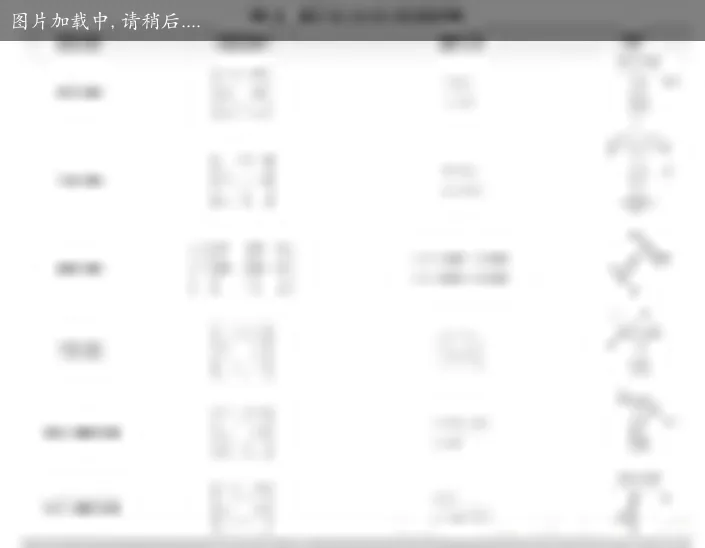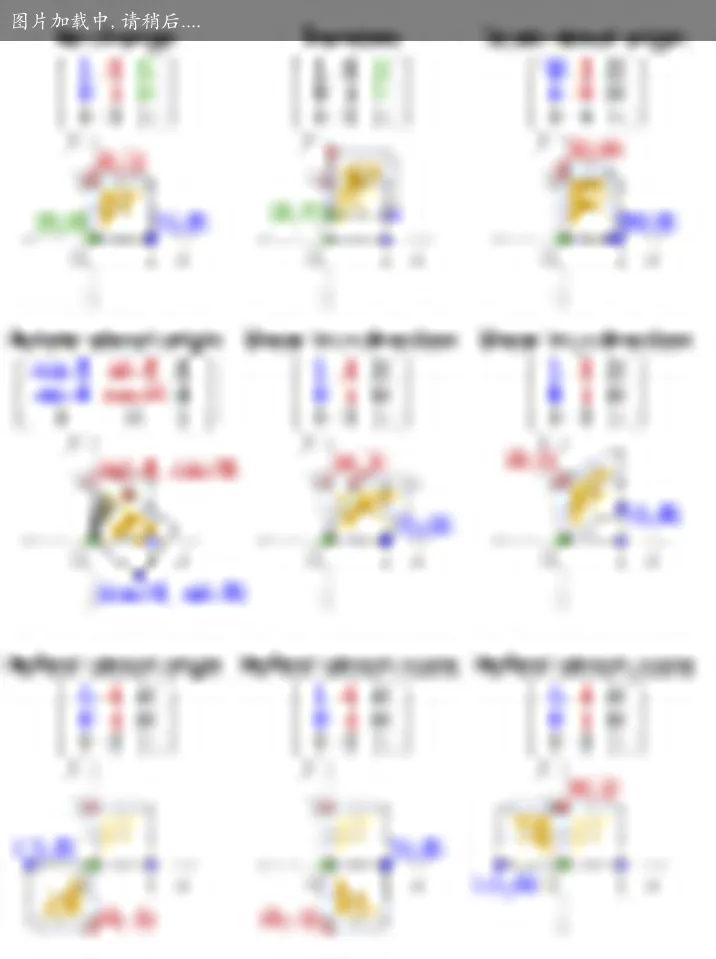#### 三维

$\left[\begin{array}{l} x^{\prime} \\ y^{\prime} \\ z^{\prime} \\ 1 \end{array}\right] = \left[\begin{array}{llll} 1 & 0 & 0 & \Delta x \\ 0 & 1 & 0 & \Delta y \\ 0 & 0 & 1 & \Delta z \\ 0 & 0 & 0 & 1 \end{array}\right] \left[\begin{array}{l} x \\ y \\ z \\ 1 \end{array}\right] = \left[\begin{array}{c} x+\Delta x \\ y+\Delta y \\ z+\Delta z \\ 1 \end{array}\right]$

## 图像边缘锐化

$\nabla f(x, y)=\left[G_{x}, G_{y}\right]^{T}=\left[\frac{\partial f}{\partial x}, \frac{\partial f}{\partial y}\right]^{T}$

$\operatorname{mag}(\nabla f)=g(x, y)=\sqrt{\frac{\partial^{2} f}{\partial x^{2}}+\frac{\partial^{2} f}{\partial y^{2}}}$

$\phi(x, y)=\arctan \left|\frac{\partial f}{\partial y} / \frac{\partial f}{\partial x}\right|$

$\begin{array}{l} G_{x}=f(x, y)-f(x-1, y) \\ G_{y}=f(x, y)-f(x, y-1) \end{array}$

### 拉普拉斯算子

$\begin{array}{l} \nabla^2 f=\frac{\partial^{2} f}{\partial x^{2}}+\frac{\partial^{2} f}{\partial y^{2}} \\ \frac{\partial^{2} f}{\partial x^{2}}=f(x+1)+f(x-1)-2 f(x) \\ \frac{\partial^{2} f}{\partial y^{2}}=f(y+1)+f(y-1)-2 f(y) \\ \end{array}$

$\nabla^2 f=[f(x+1,y)+f(x-1,y)+f(x,y+1)+f(x,y-1)]-4f(x,y)$

$g(x)= \begin{cases} f(x, y)-\nabla^{2} f(x, y) & \text{ 中心系数为负 } \\ f(x, y)+\nabla^{2} f(x, y) & \text{ 中心系数为正 } \end{cases}$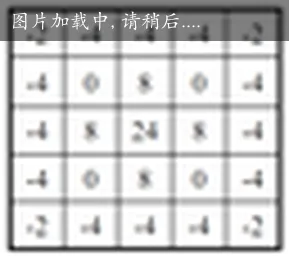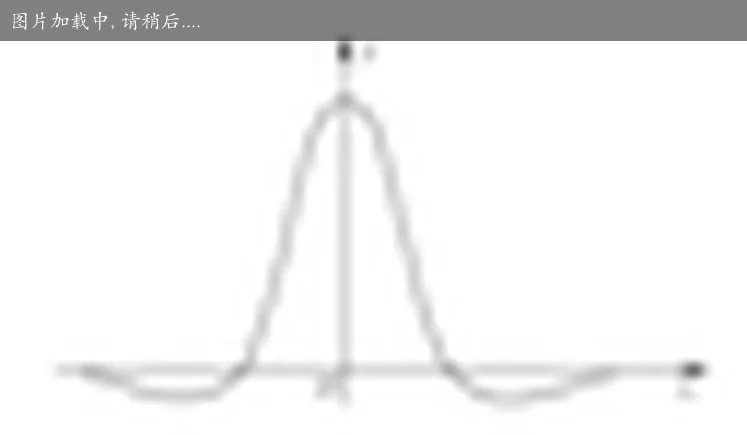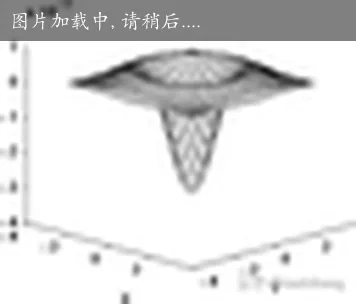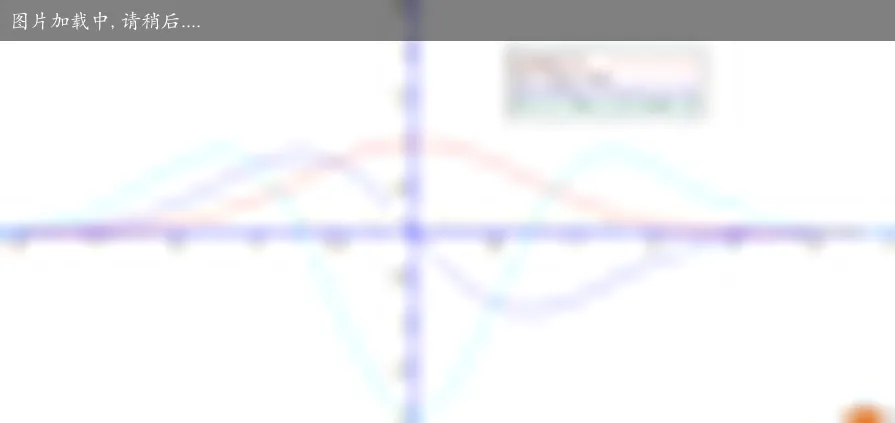### 高频提升滤波器

$g(x,y)=af(x,y)\pm \nabla f(x,y)$

### 边缘检测

$\begin{array}{|c|c|c|} \hline Z_{1} & Z_{2} & Z_{3} \\ \hline Z_{4} & Z_{5} & Z_{6} \\ \hline Z_{7} & Z_{8} & Z_{9} \\ \hline \end{array}$

#### Roberts 边缘检测算子

Robert 交叉微分算子。

$\nabla f \approx\left|z_{9}-z_{5}\right|+\left|z_{8}-z_{6}\right|$

#### Sobel 边缘检测算子

$\begin{array}{l} G_{x}=\left(Z_{7}+2 Z_{8}+Z_{9}\right)-\left(Z_{1}+2 Z_{2}+Z_{3}\right) \\ G_{y}=\left(Z_{3}+2 Z_{6}+Z_{9}\right)-\left(Z_{1}+2 Z_{4}+Z_{7}\right) \end{array}$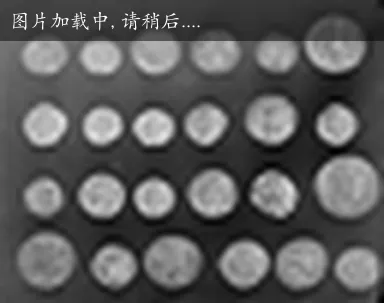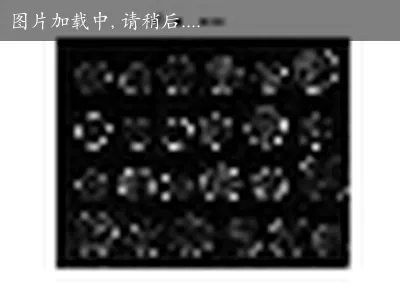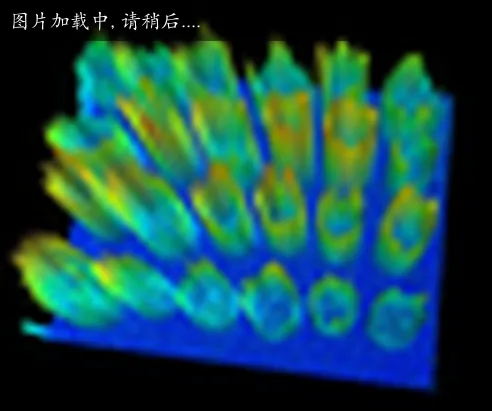#### Prewitt 边缘检测算子

$\begin{array}{l} G_{x}=\left(Z_{7}+Z_{8}+Z_{9}\right)-\left(Z_{1}+Z_{2}+Z_{3}\right) \\ G_{y}=\left(Z_{3}+Z_{6}+Z_{9}\right)-\left(Z_{1}+Z_{4}+Z_{7}\right) \end{array}$

#### Krisch 边缘检测算子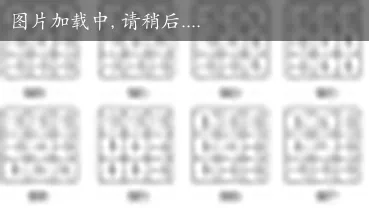#### LoG_Laplacian 高斯-拉普拉斯算子

$\left[\begin{array}{ccccc} -2 & -4 & -4 & -4 & -2 \\ -4 & 0 & 8 & 0 & -4 \\ -4 & 8 & 24 * & 8 & -4 \\ -4 & 0 & 8 & 0 & -4 \\ -2 & -4 & -4 & -4 & -2 \end{array}\right]$

## 图像分割及测量

graph LR A[图像] --> B[图像预处理] B --> | 图像分割 |C[图像识别] C --> D[图像理解] classDef orange fill:#f96; class E orange;

• 选择合适的种子点
• 确定相似性条件
• 确定停止生长的条件

### 图像的测量之投影与纹理

https://blog.csdn.net/qq_43409114/article/details/104714828

#### 衡量直方图间的相似性

• 直方图的均值：（该区域每一个像素 * 该像素的个数）/ 该区域像素点个数
• 直方图的方差：（该区域每一个像素 - 均值）^ 2 * 该像素的个数）/ 该区域像素点个数

$m_{1}=\frac{\sum z h_{1}(z)}{\sum h_{1}(z)} \quad \sigma_{1}^{2}=\frac{\sum\left(z-m_{1}\right)^{2} h_{1}(z)}{\sum h_{1}(z)}$ $m_{2}=\frac{\sum z h_{2}(z)}{\sum h_{2}(z)} \quad \sigma_{2}^{2}=\frac{\sum\left(z-m_{2}\right)^{2} h_{2}(z)}{\sum h_{2}(z)}$

#### other

$\chi_ \omega ^{2}(S,M)= \sum\limits_{i=1}^{n}{ { \omega _{i}}\frac{ { {\left( {s_{i}}-{m_{i}} \right)}^{2}}}{ { s_{i}}+{m_{i}}}}$

$D(S,M)={ { \left( \sum\limits_{i=1}^{n}{ { {\left( {s_{i}}-{m_{i}} \right)}^{2}}} \right)}^{2}}$

$D(S,M)=\frac{1}{n}\sum\limits_{i=1}^{n}{\left( 1-\frac{\left| { { s}_{i}}-{m_{i}} \right|}{\max ({ { s}_{i}},{m_{i}})} \right)}$

#### Kolmogorov-Smirnov 检测法

$\begin{array}{c} H(z)=\int_{0}^{z} h(x) d x \\ K S=\max_z \left|H_{1}(z)-H_{2}(z)\right| \end{array}$

$S D=\sum_z \left|h_{1}(z)-h_{2}(z)\right|$

#### 图像自相关函数分析法

$p(x, y)=\frac{\sum_{i=0}^{N-1} \sum_{j=0}^{N-1} f(i, j) f(i+x, j+y)}{\sum_{i=0}^{N-1} \sum_{j=0}^{N-1} f(i, j)}$ $d=\left(x^{2}+y^{2}\right)^{\frac{1}{2}}$

## 图像形态学

• 开运算（open） ：先腐蚀后膨胀的过程。开运算可以用来消除小黑点，在纤细点处分离物体、平滑较大物体的边界的同时并不明显改变其面积。

• 闭运算（close） ：先膨胀后腐蚀的过程。闭运算可以用来排除小黑洞。

• 顶帽（top-hat） ：将突出比原轮廓亮的部分。

• 黑帽（black-hat） ：将突出比原轮廓暗的部分。## 图像变换域处理

### 傅里叶变换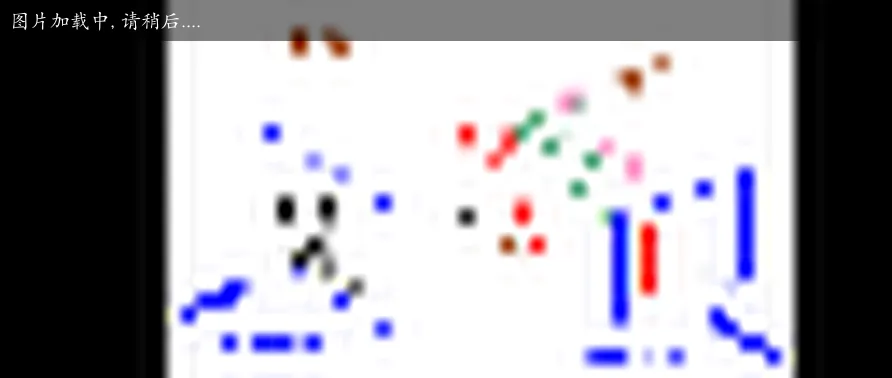$F(u) = \sum_{M=0}^{M-1} f(x) e^{-j u x \frac{2\pi} M}， u=0,1,2,\cdots,M-1$

$f(x) = {\frac 1 M} \sum_{u=0}^{M-1} F(u) e^{j u x \frac{2\pi} M}， x=0,1,2,\cdots,M-1$

$e^{jx} = \cos(x) + j \sin(x)$ $e^{-jx} = \cos(x) - j \sin(x)$ $e^{j\pi} + 1 = 0$ $\sin(x) = \frac {e^{ix} - e^{-jx}} {2i}$ $\cos(x) = \frac {e^{ix} + e^{-jx}} {2i}$

$F(u) = \sum_{M=0}^{M-1} f(x) \left( e^{-j u x \frac{2\pi} M} \right)， u=0,1,2,\cdots,M-1 \\ \Rightarrow F(u) = \sum_{M=0}^{M-1} f(x) \left( \cos(u x \frac{2\pi} M) - j \sin(u x \frac{2\pi} M) \right)， u=0,1,2,\cdots,M-1$

$f(x) = {\frac 1 M} \sum_{u=0}^{M-1} F(u) e^{j u x \frac{2\pi} M}， x=0,1,2,\cdots,M-1 \\ \Rightarrow f(x) = {\frac 1 M} \sum_{u=0}^{M-1} F(u) \left( \cos(u x \frac{2\pi} M) + j \sin(u x \frac{2\pi} M) \right) ， x=0,1,2,\cdots,M-1$

$f(x) = {\frac 1 M} \sum_{u=0}^{M-1} F(u) \left( \cos(u x \frac{2\pi} M) + j \sin(u x \frac{2\pi} M) \right) ， x=0,1,2,\cdots,M-1$

$f(x) = ( a + b j) \left( \cos(u x \frac{2\pi} M) + j \sin(u x \frac{2\pi} M) \right)$ $f(x) =\left( a\cos(u x \frac{2\pi} M) + bj\cos(u x \frac{2\pi} M) + aj \sin(u x \frac{2\pi} M) + bj\cdot j \sin(u x \frac{2\pi} M) \right)$

$f(x).real =\left( a\cos(u x \frac{2\pi} M) - b \sin(u x \frac{2\pi} M) \right)$ $f(x).imag =\left( a \sin(u x \frac{2\pi} M) + b\cos(u x \frac{2\pi} M) \right) \cdot j$

## 参考

If you have any questions or feedback, please reach out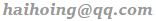.